# Chapter 9 Linear Momentum and Collisions Linear momentum

• Slides: 26
Download presentation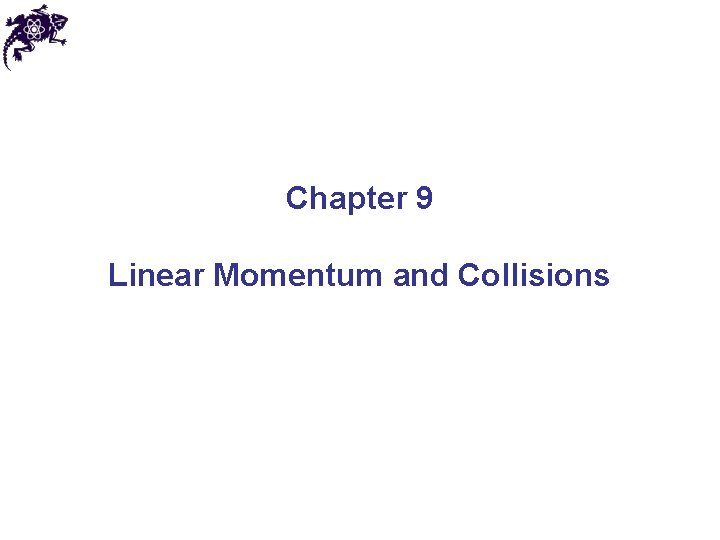Chapter 9 Linear Momentum and CollisionsLinear momentum • Linear momentum (or, simply momentum) point-like object (particle) is of a • SI unit of linear momentum is kg*m/s • Momentum is a vector, its direction coincides with the direction of velocity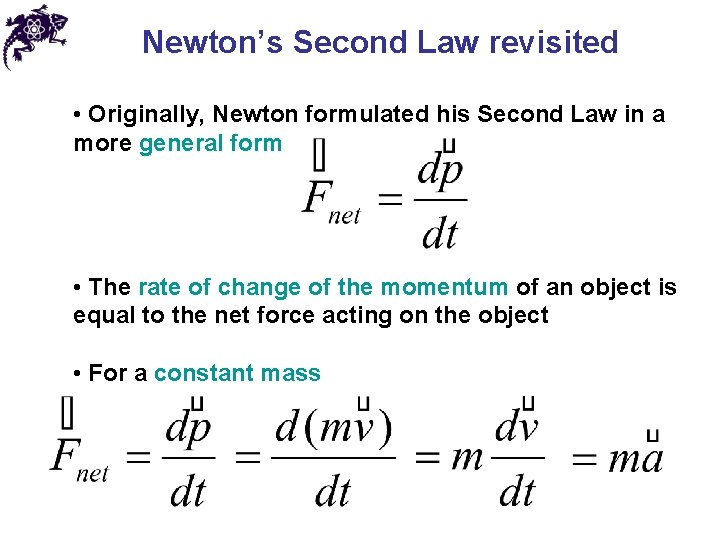Newton’s Second Law revisited • Originally, Newton formulated his Second Law in a more general form • The rate of change of the momentum of an object is equal to the net force acting on the object • For a constant mass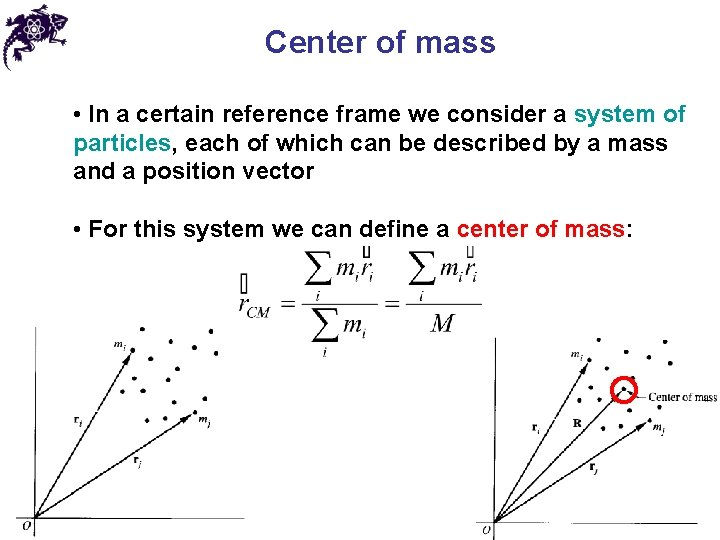Center of mass • In a certain reference frame we consider a system of particles, each of which can be described by a mass and a position vector • For this system we can define a center of mass:Center of mass of two particles • A system consists of two particles on the x axis • Then the center of mass is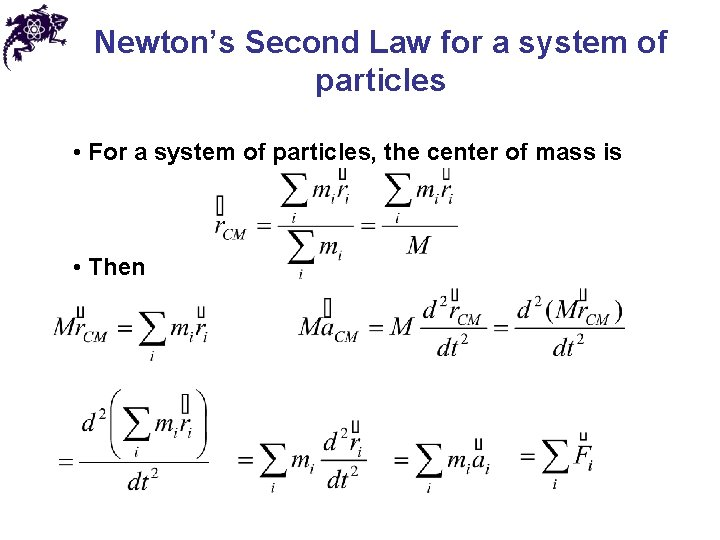Newton’s Second Law for a system of particles • For a system of particles, the center of mass is • Then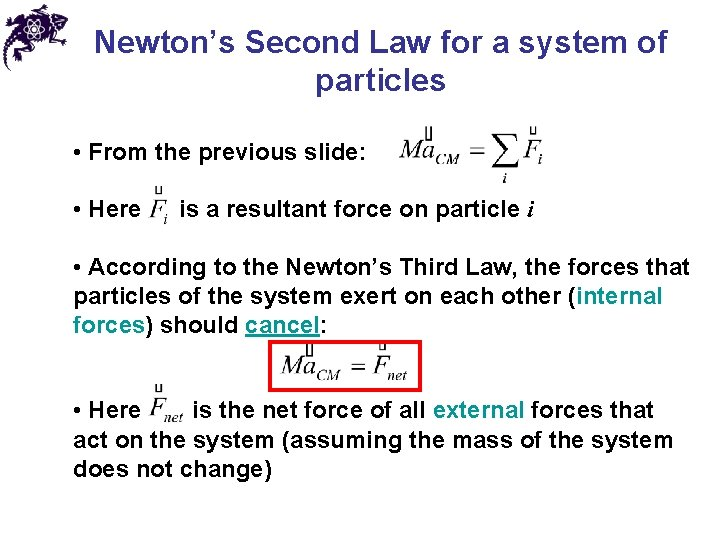Newton’s Second Law for a system of particles • From the previous slide: • Here is a resultant force on particle i • According to the Newton’s Third Law, the forces that particles of the system exert on each other (internal forces) should cancel: • Here is the net force of all external forces that act on the system (assuming the mass of the system does not change)Newton’s Second Law for a system of particles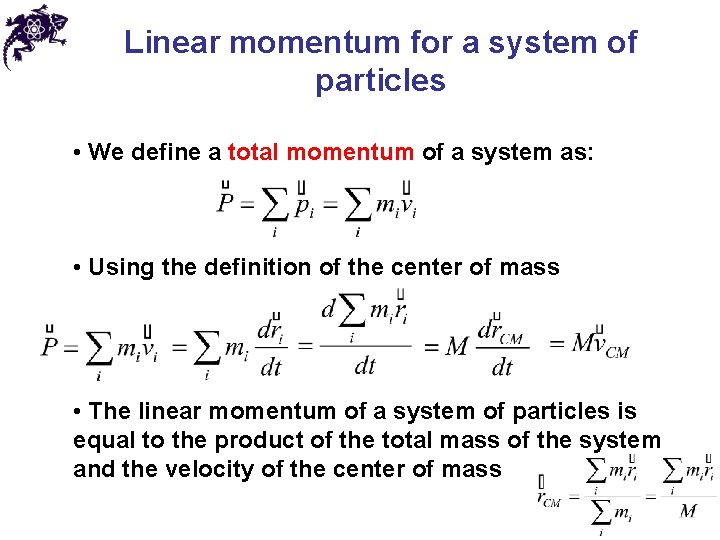Linear momentum for a system of particles • We define a total momentum of a system as: • Using the definition of the center of mass • The linear momentum of a system of particles is equal to the product of the total mass of the system and the velocity of the center of massLinear momentum for a system of particles • Total momentum of a system: • Taking a time derivative • Alternative form of the Newton’s Second Law for a system of particlesConservation of linear momentum • From the Newton’s Second Law • If the net force acting on a system is zero, then • If no net external force acts on a system of particles, the total linear momentum of the system is conserved (constant) • This rule applies independently to all components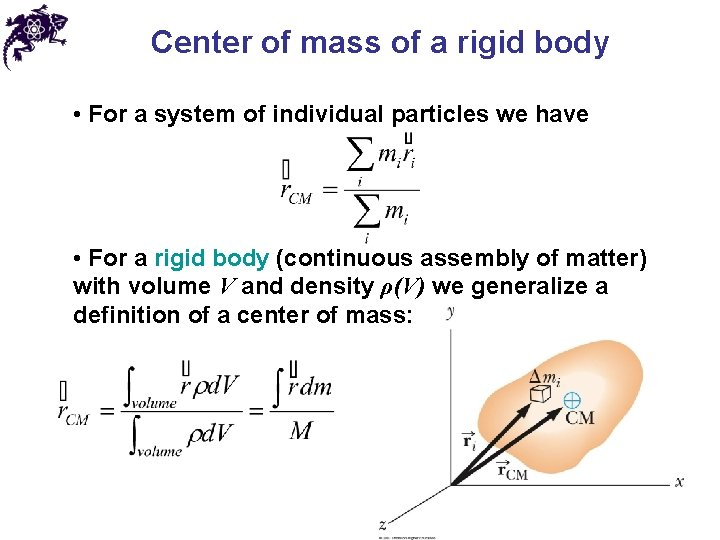Center of mass of a rigid body • For a system of individual particles we have • For a rigid body (continuous assembly of matter) with volume V and density ρ(V) we generalize a definition of a center of mass: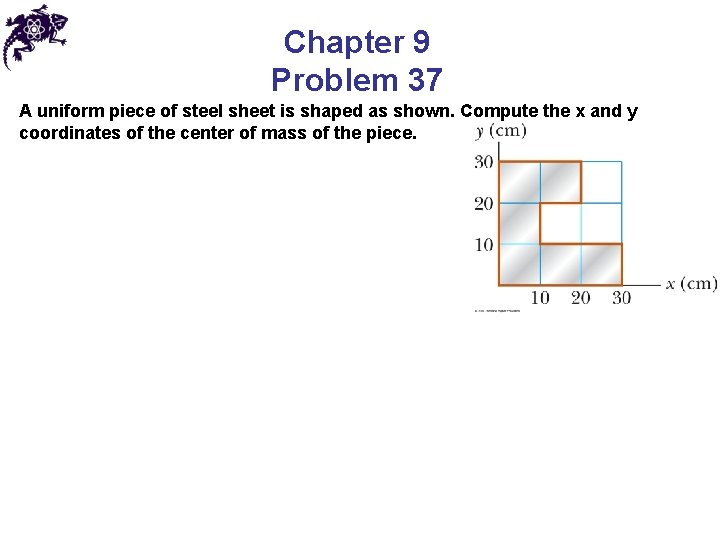Chapter 9 Problem 37 A uniform piece of steel sheet is shaped as shown. Compute the x and y coordinates of the center of mass of the piece.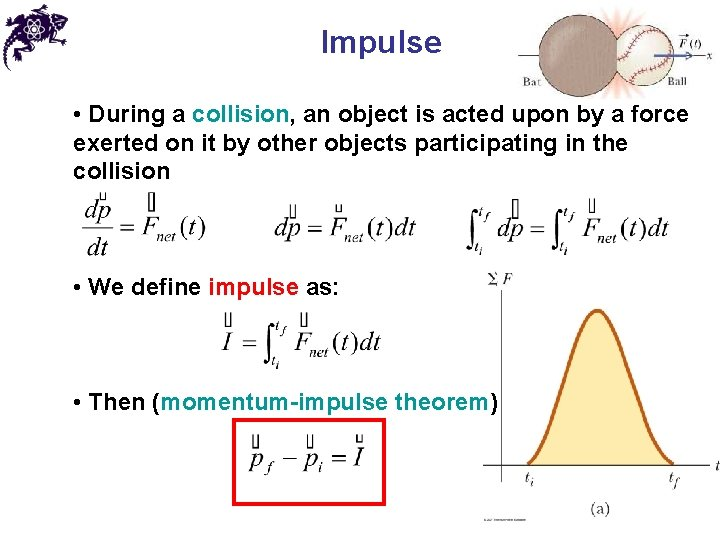Impulse • During a collision, an object is acted upon by a force exerted on it by other objects participating in the collision • We define impulse as: • Then (momentum-impulse theorem)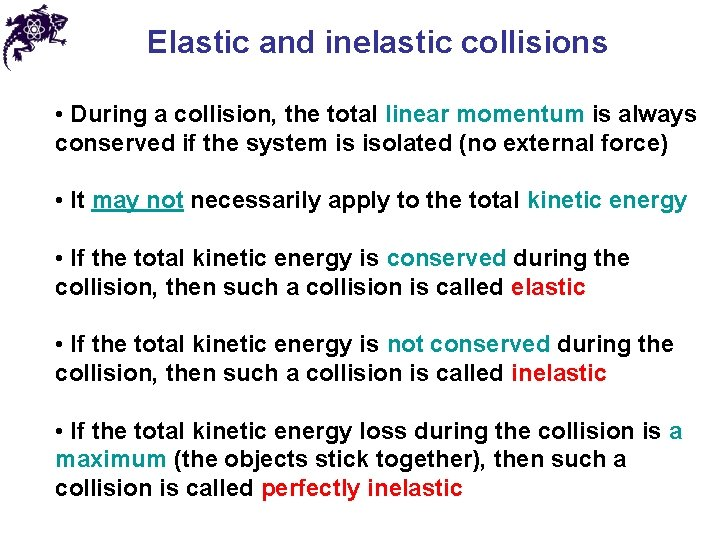Elastic and inelastic collisions • During a collision, the total linear momentum is always conserved if the system is isolated (no external force) • It may not necessarily apply to the total kinetic energy • If the total kinetic energy is conserved during the collision, then such a collision is called elastic • If the total kinetic energy is not conserved during the collision, then such a collision is called inelastic • If the total kinetic energy loss during the collision is a maximum (the objects stick together), then such a collision is called perfectly inelasticElastic collision in 1 D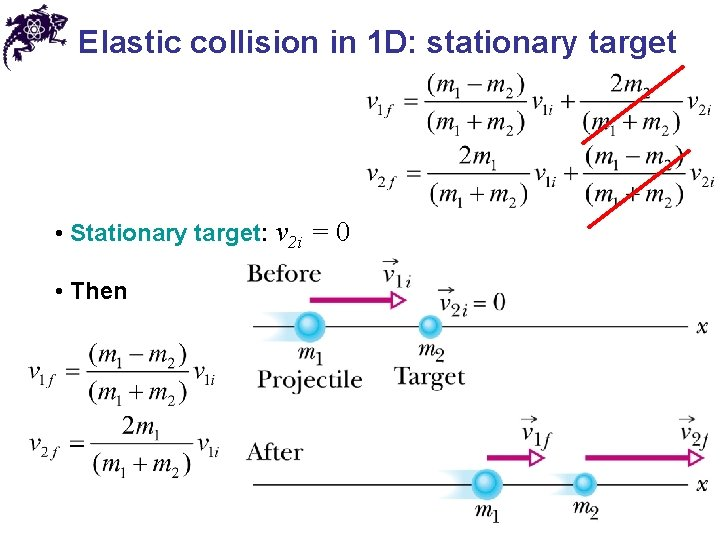Elastic collision in 1 D: stationary target • Stationary target: v 2 i = 0 • Then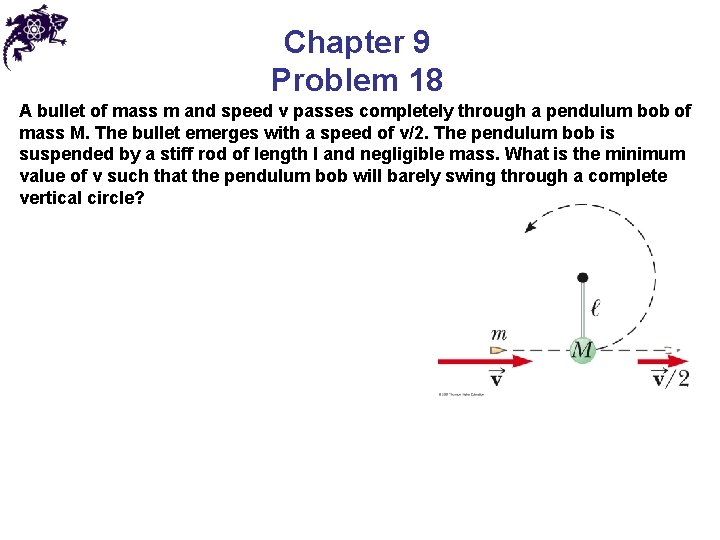Chapter 9 Problem 18 A bullet of mass m and speed v passes completely through a pendulum bob of mass M. The bullet emerges with a speed of v/2. The pendulum bob is suspended by a stiff rod of length l and negligible mass. What is the minimum value of v such that the pendulum bob will barely swing through a complete vertical circle?Perfectly inelastic collision in 1 DCollisions in 2 D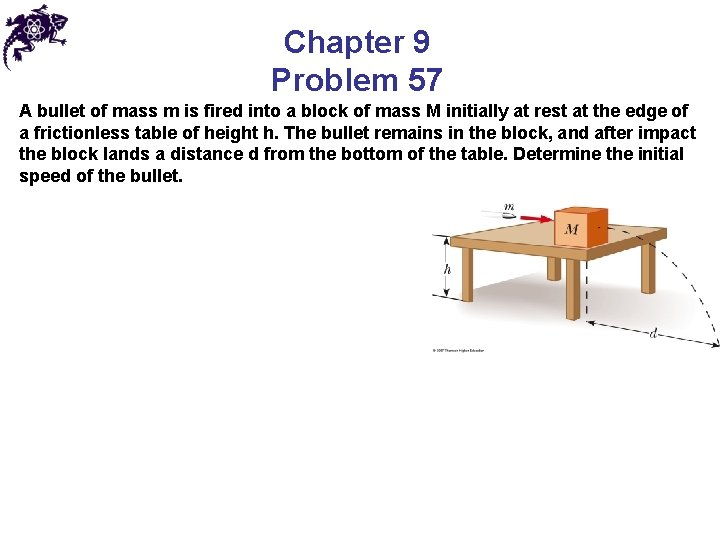Chapter 9 Problem 57 A bullet of mass m is fired into a block of mass M initially at rest at the edge of a frictionless table of height h. The bullet remains in the block, and after impact the block lands a distance d from the bottom of the table. Determine the initial speed of the bullet.Answers to the even-numbered problems Chapter 9 Problem 8 1. 39 kg⋅m/s upwardAnswers to the even-numbered problems Chapter 9 Problem 16 (a) 2. 50 m/s; (b) 3. 75 × 104 J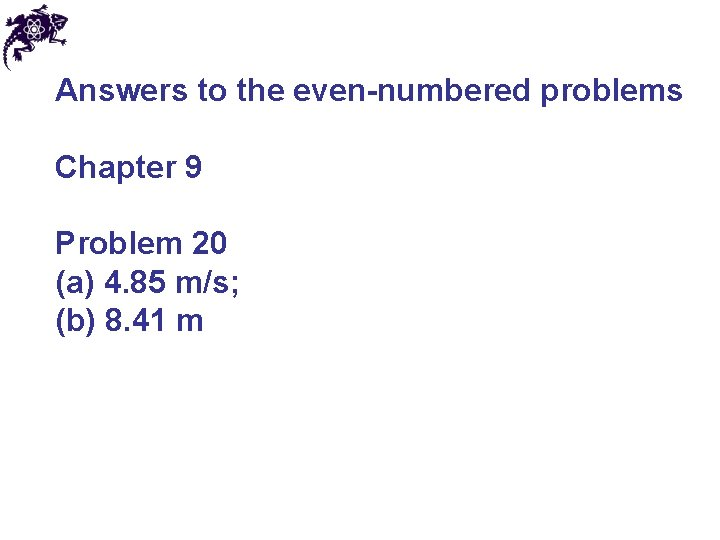Answers to the even-numbered problems Chapter 9 Problem 20 (a) 4. 85 m/s; (b) 8. 41 m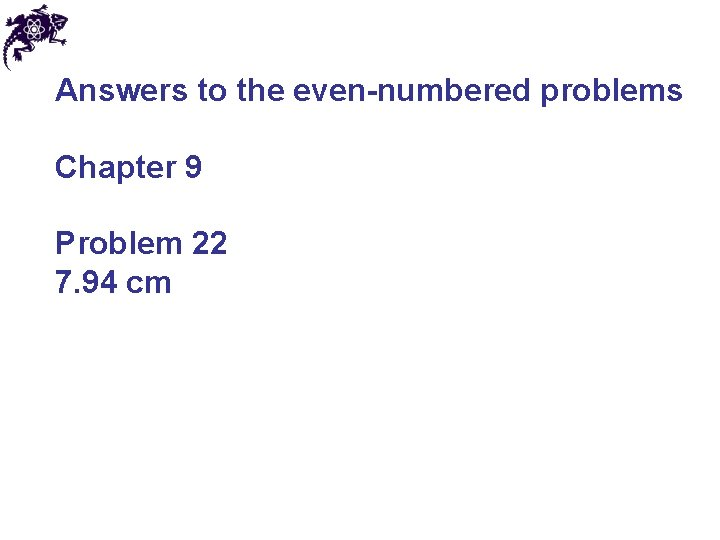Answers to the even-numbered problems Chapter 9 Problem 22 7. 94 cmAnswers to the even-numbered problems Chapter 9 Problem 58 (a) – 0. 667 m/s; (b) 0. 952 m